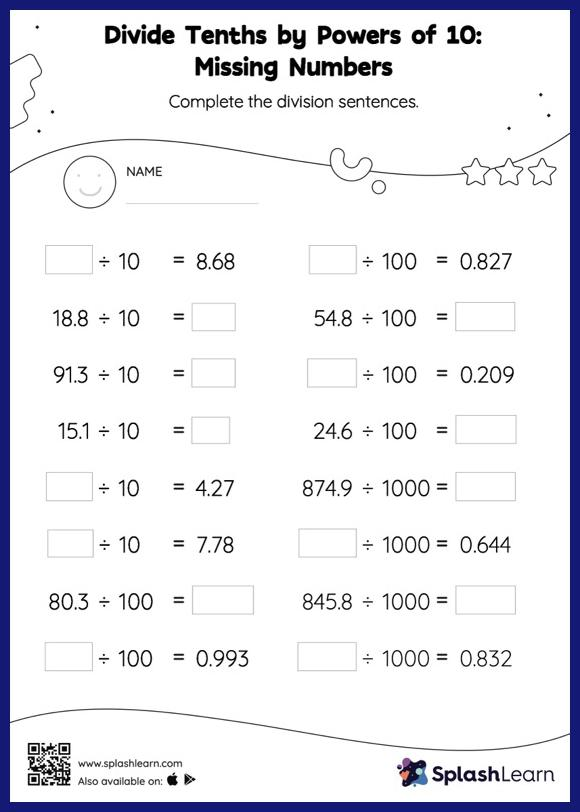# Divide Tenths by Powers of 10: Missing Numbers Worksheet

Home > Divide Tenths by Powers of 10: Missing NumbersWhen a decimal is divided by a power of 10, the decimal point moves to the left by the same amount as the number of zeros in the power of 10. This concept helps students find the missing number in divide tenths by powers of 10 worksheet. This worksheet is about practicing with the horizontal format in which numbers are written side by side. To develop flexibility with numbers and operations, students need to have enough practice in this format and not just rely on the vertical/column method.